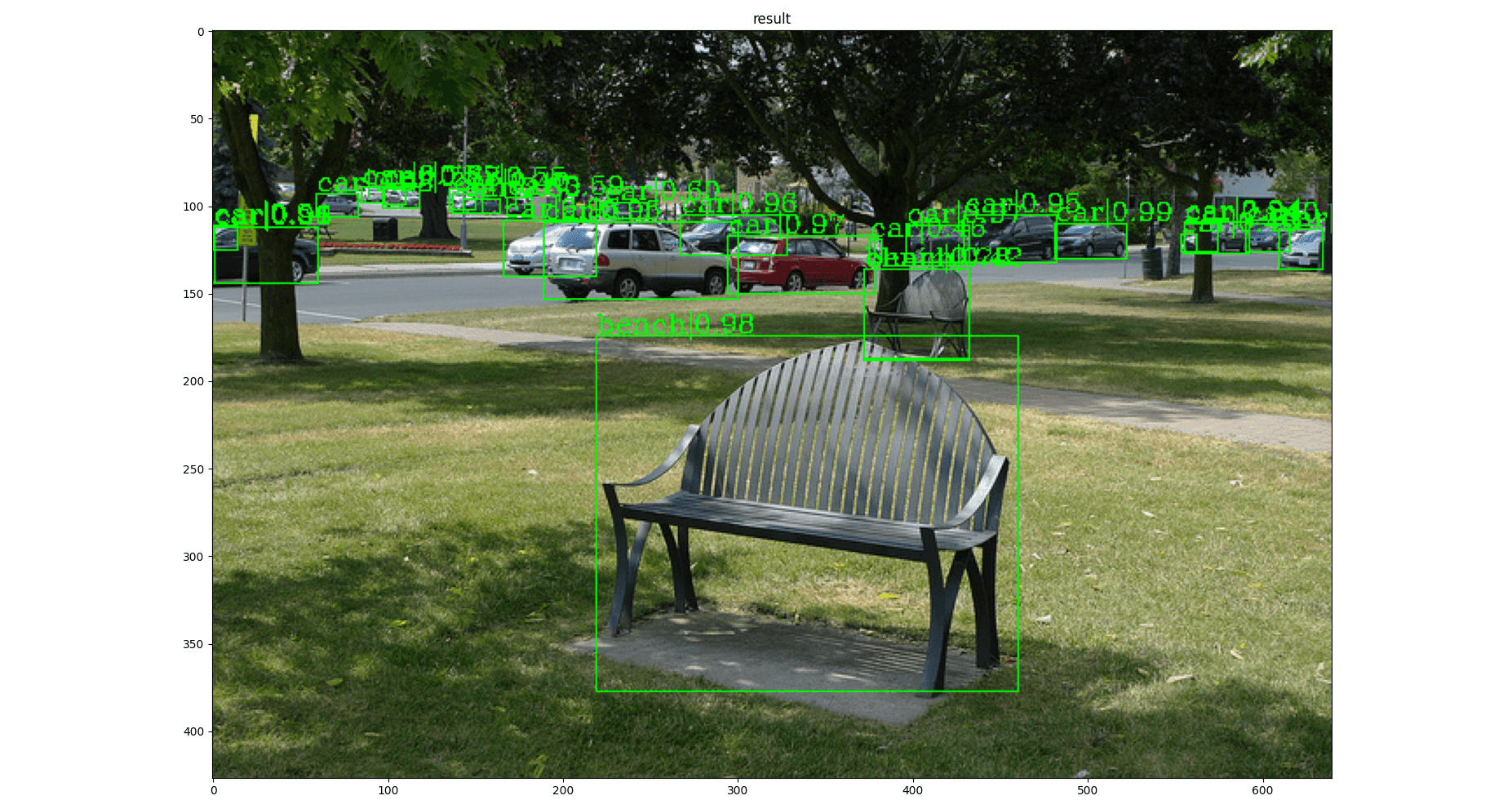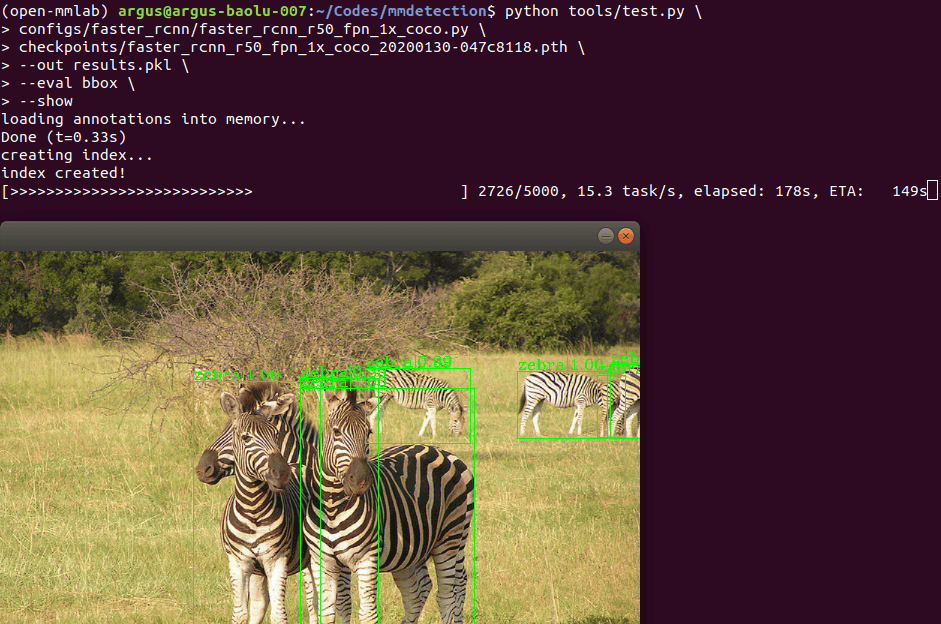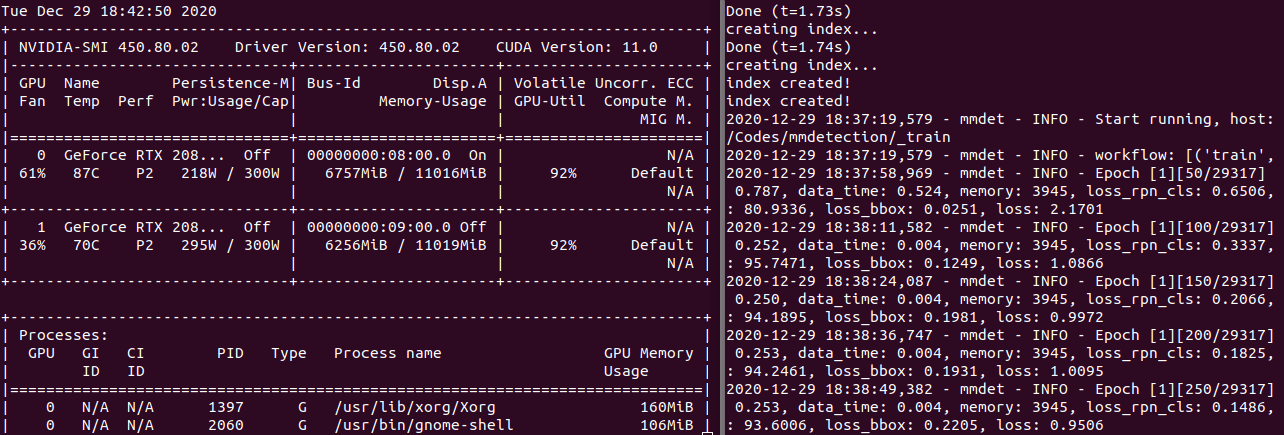# MMDetection 开始

## 环境准备#

### 开发环境#

# 创建 Python 虚拟环境
conda create -n open-mmlab python=3.7 -y
conda activate open-mmlab
# 安装 PyTorch with CUDA
conda install pytorch==1.6.0 torchvision==0.7.0 cudatoolkit=10.2 -c pytorch -y
# 安装 MMCV
# 安装 MMDetection
git clone https://github.com/open-mmlab/mmdetection.git
cd mmdetection
pip install -r requirements/build.txt
pip install -v -e .

`pytorch==1.7.0` 时多卡训练会发生问题，需参考此 Issue。命令参考：

conda install pytorch==1.7.0 torchvision==0.8.1 cudatoolkit=10.2 -c pytorch -y

## 现有模型进行推断#

### Faster RCNN#

R-50-FPNpytorch1x4.021.437.4configmodel | log

conda activate open-mmlab
cd mmdetection/
python demo/image_demo.py \
demo/demo.jpg \
configs/faster_rcnn/faster_rcnn_r50_fpn_1x_coco.py \
checkpoints/faster_rcnn_r50_fpn_1x_coco_20200130-047c8118.pth## 现有模型进行测试#

### 准备数据集#

mmdetection
├── data
│ ├── coco
│ │ ├── annotations
│ │ ├── train2017
│ │ ├── val2017
│ │ ├── test2017

### 测试现有模型#

cd mmdetection/
# single-gpu testing
python tools/test.py \
configs/faster_rcnn/faster_rcnn_r50_fpn_1x_coco.py \
checkpoints/faster_rcnn_r50_fpn_1x_coco_20200130-047c8118.pth \
--out results.pkl \
--eval bbox \
--show
# multi-gpu testing
bash tools/dist_test.sh \
configs/faster_rcnn/faster_rcnn_r50_fpn_1x_coco.py \
checkpoints/faster_rcnn_r50_fpn_1x_coco_20200130-047c8118.pth \
2 \
--out results.pkl \
--eval bboxDone (t=0.33s)
creating index...
index created!
[>>>>>>>>>>>>>>>>>>>>>>>>>>>>>>>>>>>>>>>>>>>>>>>>>>] 5000/5000, 15.3 task/s, elapsed: 328s, ETA: 0s
writing results to results.pkl
Evaluating bbox...
DONE (t=0.89s)
creating index...
index created!
Running per image evaluation...
Evaluate annotation type *bbox*
DONE (t=26.17s).
Accumulating evaluation results...
DONE (t=4.10s).
Average Precision (AP) @[ IoU=0.50:0.95 | area= all | maxDets=100 ] = 0.374
Average Precision (AP) @[ IoU=0.50 | area= all | maxDets=1000 ] = 0.581
Average Precision (AP) @[ IoU=0.75 | area= all | maxDets=1000 ] = 0.404
Average Precision (AP) @[ IoU=0.50:0.95 | area= small | maxDets=1000 ] = 0.212
Average Precision (AP) @[ IoU=0.50:0.95 | area=medium | maxDets=1000 ] = 0.410
Average Precision (AP) @[ IoU=0.50:0.95 | area= large | maxDets=1000 ] = 0.481
Average Recall (AR) @[ IoU=0.50:0.95 | area= all | maxDets=100 ] = 0.517
Average Recall (AR) @[ IoU=0.50:0.95 | area= all | maxDets=300 ] = 0.517
Average Recall (AR) @[ IoU=0.50:0.95 | area= all | maxDets=1000 ] = 0.517
Average Recall (AR) @[ IoU=0.50:0.95 | area= small | maxDets=1000 ] = 0.326
Average Recall (AR) @[ IoU=0.50:0.95 | area=medium | maxDets=1000 ] = 0.557
Average Recall (AR) @[ IoU=0.50:0.95 | area= large | maxDets=1000 ] = 0.648
OrderedDict([('bbox_mAP', 0.374), ('bbox_mAP_50', 0.581), ('bbox_mAP_75', 0.404), ('bbox_mAP_s', 0.212), ('bbox_mAP_m', 0.41), ('bbox_mAP_l', 0.481), ('bbox_mAP_copypaste', '0.374 0.581 0.404 0.212 0.410 0.481')])

## 标准数据集训练模型#

### 准备配置文件#

• `lr=0.005` for 2 GPUs * 2 imgs/gpu
• `lr=0.01` for 4 GPUs * 2 imgs/gpu
• `lr=0.02` for 8 GPUs and 2 img/gpu (batch size = 8*2 = 16), DEFAULT
• `lr=0.08` for 16 GPUs * 4 imgs/gpu
_base_ = [
'../_base_/models/faster_rcnn_r50_fpn.py',
'../_base_/datasets/coco_detection.py',
'../_base_/schedules/schedule_1x.py', '../_base_/default_runtime.py'
]
# optimizer
optimizer = dict(type='SGD', lr=0.005, momentum=0.9, weight_decay=0.0001)

### 训练模型#

cd mmdetection/
# single-gpu training
python tools/train.py \
configs/faster_rcnn/faster_rcnn_r50_fpn_1x_coco.py \
--work-dir _train
# multi-gpu training
bash ./tools/dist_train.sh \
configs/faster_rcnn/faster_rcnn_r50_fpn_1x_coco.py \
2 \
--work-dir _train## 自定义数据集训练模型#

### 自定义数据集#

conda activate open-mmlab
# Dataset Management Framework (Datumaro)
pip install 'git+https://github.com/openvinotoolkit/datumaro'
# pip install tensorflow
datum convert --input-format voc --input-path ~/datasets/VOC2012 \
--output-format coco --output-dir ~/datasets/coco_voc2012_cat \
--filter '/item[annotation/label="cat"]'

### 准备配置文件#

# The new config inherits a base config to highlight the necessary modification
_base_ = [
'../_base_/models/faster_rcnn_r50_fpn.py',
'../_base_/datasets/coco_detection.py',
'../_base_/schedules/schedule_1x.py', '../_base_/default_runtime.py'
]
# We also need to change the num_classes in head to match the dataset's annotation
model = dict(
# Modify dataset related settings
dataset_type = 'COCODataset'
classes = ('cat',)
data_root = '/home/john/datasets/'
data = dict(
train=dict(
img_prefix=data_root + 'VOC2012/JPEGImages/',
classes=classes,
ann_file=data_root + 'coco_voc2012_cat/annotations/instances_train.json'),
val=dict(
img_prefix=data_root + 'VOC2012/JPEGImages/',
classes=classes,
ann_file=data_root + 'coco_voc2012_cat/annotations/instances_val.json'),
test=dict(
img_prefix=data_root + 'VOC2012/JPEGImages/',
classes=classes,
ann_file=data_root + 'coco_voc2012_cat/annotations/instances_val.json'))
evaluation = dict(interval=100)
# Modify schedule related settings
optimizer = dict(type='SGD', lr=0.005, momentum=0.9, weight_decay=0.0001)
total_epochs = 10000
# Modify runtime related settings
checkpoint_config = dict(interval=10)
# We can use the pre-trained model to obtain higher performance
• `model` 配置 `num_classes=1` 为类别数量
• `dataset` 配置为准备的自定义数据集
• `schedule` 配置训练的 `lr` 及迭代轮次 `total_epochs`
• `runtime` 可配置 `checkpoint` 间隔多少存一个。默认 1 epoch 1 个，空间不够用😶

### 训练模型#

# single-gpu training
python tools/train.py \
configs/voc_cat/faster_rcnn_r50_fpn_1x_voc_cat.py \
--work-dir _train_voc_cat
# multi-gpu training
bash ./tools/dist_train.sh \
configs/voc_cat/faster_rcnn_r50_fpn_1x_voc_cat.py \
2 \
--work-dir _train_voc_cat

bash ./tools/dist_train.sh \
configs/voc_cat/faster_rcnn_r50_fpn_1x_voc_cat.py \
2 \
--work-dir _train_voc_cat \
--resume-from _train_voc_cat/epoch_100.pth

pip uninstall pycocotools mmpycocotools
pip install mmpycocotools

### 查看训练 loss#

pip install seaborn
python tools/analyze_logs.py plot_curve \
_train_voc_cat/*.log.json \
--keys loss_cls loss_bbox \
--legend loss_cls loss_bbox

### 测试模型#

# single-gpu testing
python tools/test.py \
configs/voc_cat/faster_rcnn_r50_fpn_1x_voc_cat.py \
_train_voc_cat/latest.pth \
--out results.pkl \
--eval bbox \
--show
# multi-gpu testing
bash tools/dist_test.sh \
configs/voc_cat/faster_rcnn_r50_fpn_1x_voc_cat.py \
_train_voc_cat/latest.pth \
2 \
--out results.pkl \
--eval bbox